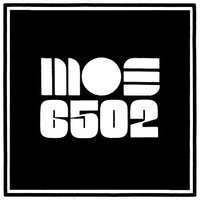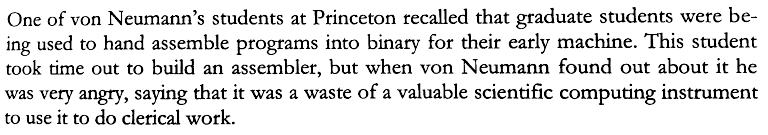## 6502 Assembly is the language used to program the Famicom, BBC Micro and Commodore 64 computers.

Assembly is any low-level programming language in which there is a very strong correspondence between the instructions in the language and the architecture's machine code instructions. An assembler translates the assembly language syntax into their numerical equivalents.

A programming language is low level when its programs require attention to the irrelevant.— Typical Assembly Programmer

This page focuses on the assembly language for the 6502 processor, targetting the Famicom. Prior to learning assembly, I would recommend learning the basics of binary numbers. To explore a system with minimal instructions set, try the Uxn virtual machine. To see an example of a complete 6502 assembly project, see Spacetime.### Lexicon

Directives are commands you send to the assembler to do things like locating code in memory. They start with . and are indented. This sample directive tells the assembler to put the code starting at memory location \$8000, which is inside the game ROM area. Labels are aligned to the far left and have a : at the end. The label is just something you use to organize your code and make it easier to read. The assembler translates the label into an address.

Opcodes are the instructions that the processor will run, and are indented like the directives. In this sample, JMP is the opcode that tells the processor to jump to the MyFunction label. Operands are additional information for the opcode. Opcodes have between one and three operands. In this example the #\$FF is the operand:

Comments are to help you understand in English what the code is doing. When you write code and come back later, the comments will save you. You do not need a comment on every line, but should have enough to explain what is happening. Comments start with a ; and are completely ignored by the assembler. They can be put anywhere horizontally, but are usually spaced beyond the long lines.

```  .org \$8000
MyFunction:                    ; A comment
LDA #\$FF
JMP MyFunction
```

### Styleguide

Major comments are prefixed with two semi-colons, and minor comments are found at the end of a line on the 32nd column if available. Variables and subroutines are lowercase, constants and vectors are uppercase, and routines are capitalized.

```;; Variables

.enum \$0000                  ; Zero Page variables
pos_x                   .dsb 1
pos_y                   .dsb 1
.ende

;; Constants

SPRITE_Y            .equ \$0200
SPRITE_X            .equ \$0203

RESET:
NOP

Forever:
JMP Forever

NMI:
RTI

;; Routines

Check_Collision:
LDA pos_y
CMP #\$88                     ; Floor is at 32y
BCC @done
LDA #\$88
STA pos_y
@done:
RTS

;; Tables

Table_Name:
.db \$40,\$46,\$4c,\$52,\$58,\$5e,\$63,\$68

;; Vectors

.dw NMI
.dw RESET
.dw 0
.incbin "src/sprite.chr"
```

The lin6 linter is used to enfore this style on the various assembly projects found on this site.

### Registers

The 6502 handles data in its registers, each of which holds one byte(8 bits) of data. There are a total of three general use and two special purpose registers:

Note: When you use X it adds the value of X to the memory address and uses the 16-bit value at that address to do the write. Whereas when you use Y it adds the value of Y to the address stored in the memory address it's reading from instead. 6502 is little-endian, so \$0200 is stored as \$00 \$02 in memory.

• A: The accumulator handles all arithmetic and logic. The real heart of the system..
• X&Y: General purpose registers with limited abilities..
• SP: The stack pointer is decremented every time a byte is pushed onto the stack, and incremented when a byte is popped off the stack..
• PC: The program counter is how the processor knows at what point in the program it currently is. It’s like the current line number of an executing script. In the JavaScript simulator the code is assembled starting at memory location \$0600, so PC always starts there..
• PF: The Processor flag contains 7 bits, each flag live in a single bit. The flags are set by the processor to give information about the previous instruction.

The 6502 has 9 major(13 in total) addressing modes, or ways of accessing memory.

### Common Opcodes

LDA #\$0ALoaD the value 0A into the accumulator A. The number part of the opcode can be a value or an address. If the value is zero, the zero flag will be set.
LDX \$0000LoaD the value at address \$0000 into the index register X. If the value is zero, the zero flag will be set.
LDY #\$FF LoaD the value \$FF into the index register Y. If the value is zero, the zero flag will be set.
STA \$2000STore the value from accumulator A into the address \$2000. The number part must be an address.
STX \$4016STore value in X into \$4016. The number part must be an address.
STY \$0101STore Y into \$0101. The number part must be an address.
TAX Transfer the value from A into X. If the value is zero, the zero flag will be set.
TAY Transfer A into Y. If the value is zero, the zero flag will be set.
TXA Transfer X into A. If the value is zero, the zero flag will be set.
TYA Transfer Y into A. If the value is zero, the zero flag will be set.
Math opcodes
ADC #\$01 ADd with Carry. A = A + \$01 + carry. If the result is zero, the zero flag will be set
SBC #\$80 SuBtract with Carry. A = A - \$80 - (1 - carry). If the result is zero, the zero flag will be set
CLC CLear Carry flag in status register. Usually this should be done before ADC
SEC SEt Carry flag in status register. Usually this should be done before SBC
INC \$0100INCrement value at address \$0100. If the result is zero, the zero flag will be set
DEC \$0001DECrement \$0001. If the result is zero, the zero flag will be set
INY INcrement Y register. If the result is zero, the zero flag will be set
INX INcrement X register. If the result is zero, the zero flag will be set
DEY DEcrement Y. If the result is zero, the zero flag will be set
DEX DEcrement X. If the result is zero, the zero flag will be set
ASL A Arithmetic Shift Left. Shift all bits one position to the left. This is a multiply by 2. If the result is zero, the zero flag will be set
LSR \$6000Logical Shift Right. Shift all bits one position to the right. This is a divide by 2. If the result is zero, the zero flag will be set
Comparison opcodes
CMP #\$01 CoMPare A to the value \$01. This actually does a subtract, but does not keep the result. Instead you check the status register to check for equal, . Less than, or greater than
CPX \$0050ComPare X to the value at address \$0050
CPY #\$FF ComPare Y to the value \$FF
Control-Flow opcodes
BEQ \$FF00Branch if EQual, contnue running code there. First you would do a CMP, which clears or sets the zero flag. Then the BEQ will check the zero flag. If zero is set (values were equal) the code jumps to \$FF00 and runs there. If zero is clear (values not equal) there is no jump, runs next instruction
BNE \$FF00Branch if Not Equal - opposite above, jump is made when zero flag is clear### Compare

The compare instructions set or clear three of the status flags (Carry, Zero, and Negative) that can be tested with branch instructions, without altering the contents of the operand. There are three types of compare instructions:

 Instruction Description CMP Compare Memory and Accumulator CPX Compare Memory and IndexX CPY Compare Memory and Index Y

The CMP instruction supports eight different addressing modes, the same ones supported by the ADC and SBC instructions. Since the X and Y registers function primarily as counters and indexes, the CPX and CPY instructions do not require this elaborate addressing capability and operate with just three addressing modes (immediate, absolute, and zero page).

The compare instructions subtract (without carry) an immediate value or the contents of a memory location from the addressed register, but do not save the result in the register. The only indications of the results are the states of the three status flags: Negative (N), Zero (Z), and Carry (C). The combination of these three flags indicate whether the register contents are less than, equal to (the same as), or greater than the operand "data" (the immediate value or contents of the addressed memory location. The table below summarizes the result indicators for the compare instructions.

 Compare Result N Z C A, X, or Y < Memory * 0 0 A, X, or Y = Memory 0 1 1 A, X, or Y > Memory * 0 1

The compare instructions serve only one purpose; they provide information that can be tested by a subsequent branch instruction. For example, to branch if the contents of a register are less than an immediate or memory value, you would follow the compare instruction with a Branch on Carry Clear (BCC) instruction, as shown by the following:

### Comparing Memory to the Accumulator

```  CMP  \$20    ; Accumulator less than location \$20?
BCC  THERE
HERE:
; No, continue execution here.
THERE:
; Execute this if Accumulator is less than location \$20.
```

### Use of Branch Instructions with Compare

 To Branch If Follow compare instruction with For unsigned numbers For signed numbers Register is less than data BCC THERE BMI THERE Register is equal to data BEQ THERE BEQ THERE Register is greater than data BEQ HEREBCS THERE BEQ HEREBPL THERE Register is less than or equal to data BCC THEREBEQ THERE BMI THEREBEQ THERE Register is greater than or equal to data BCS THERE BPL THERE

### Math

#### Modulo

Returns in register A.

```Mod:
LDA \$00  ; memory addr A
SEC
Modulus:
SBC \$01  ; memory addr B
BCS Modulus
RTS
```

#### Division

Rounds up, returns in register A.

```Div:
LDA \$00 ; memory addr A
LDX #0
SEC
Divide:
INX
SBC \$01 ; memory addr B
BCS Divide
TXA
RTS
```